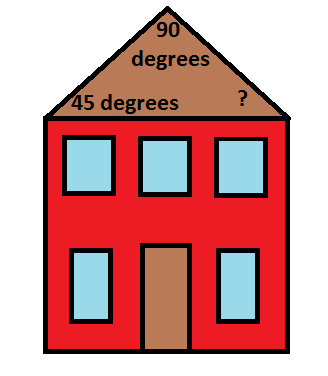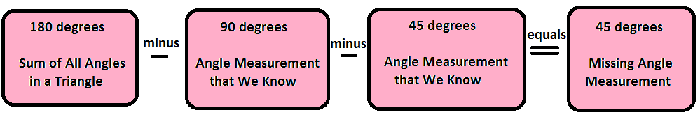# Triangle Sum Theorem: Definition & Examples

Instructor: Allison Petrovic

Allison has experience teaching high school and college mathematics and has a master's degree in mathematics education.

In this lesson, we will learn about the Triangle Sum Theorem. We will also look at how useful the Triangle Sum Theorem is when looking for missing angle measurements inside of a triangle.

## Building a House!

Imagine that you are a construction worker and planning your next home to build. Working on the roof, you are trying to figure out all of the measurements. One part of the roof that you are looking at is in the shape of a triangle. You know it is a triangle because it is a shape with 3 sides and 3 angles. You already know that one of the angle measurements is 45 degrees. The second given angle is a right angle. Remember that a right angle is an angle that is 90 degrees. You need to figure out the third angle measurement. You are happy because you remember learning the Triangle Sum Theorem from math class!## Triangle Sum Theorem

The Triangle Sum Theorem states that if you add all three interior angles, those are the angles inside the triangle, they would add up to 180 degrees. It is easy to remember that we add the three angle measurements to get 180 degrees because of the word sum in the name of the theorem. To make it short and sweet: a + b + c = 180°

In our problem, we need to determine the third angle measurement. To find this angle measurement, all we have to do is subtract the two angle measurements that we have from 180 degrees. So, we have the following:By using the Triangle Sum Theorem, we can say that the missing angle measurement is 45 degrees!

## Example 1

Let's try another example. Suppose we have a triangle with two known measurements. One of the angle measurements is 30 degrees. The second angle measurement is 60 degrees. We need to find the measurement of the third angle. Let's use the Triangle Sum Theorem to help us solve our problem! We know that the sum of all the angles inside a triangle is 180 degrees. We have two of the three angle measurements. So, all we have to do is subtract the two angle measurements that we have from 180 degrees.

180 degrees - 60 degrees - 30 degrees = 90 degrees

We can say that the third angle measurement is 90 degrees! We have a right angle!

To unlock this lesson you must be a Study.com Member.

### Register to view this lesson

Are you a student or a teacher?

#### See for yourself why 30 million people use Study.com

##### Become a Study.com member and start learning now.
Back
What teachers are saying about Study.com

### Earning College Credit

Did you know… We have over 200 college courses that prepare you to earn credit by exam that is accepted by over 1,500 colleges and universities. You can test out of the first two years of college and save thousands off your degree. Anyone can earn credit-by-exam regardless of age or education level.